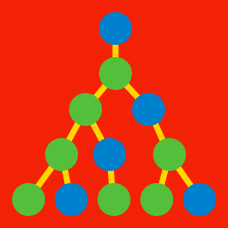Probability

Linear Recurrence Relations - Calculating Initial Terms

Let $\{x_n\}$ be the sequence $1, 5, 9, 13, \ldots.$

If $\{x_n\}$ can be defined as the recurrence relation $x_7 = a, \quad x_{n+1} = x_n + b,$ find the value of $a + b.$

The sequence $X$ is defined by $X_1 = 2$ and $X_n = X_{n-1} + 3$ for $n \geq 2.$ What is the value of $X_{10}?$

A sequence $V$ satisfies $V_1 = 5, V_2 = 2$ and $V_n = V_{n-1} + V_{n-2}$ for $n \geq 2.$ What is the value of $V_6?$

In the sequence $a_{n},$ if $a_{1} = 1, a_{2}= 5$ and $2a_{k+1} = a_{k} + a_{k+2},$ what is $a_{10}?$

The sequence $F$ is defined by $F_1 = 1$ and $F_n = 2F_{n-1}$ for $n \geq 2.$ What is $F_6?$

×